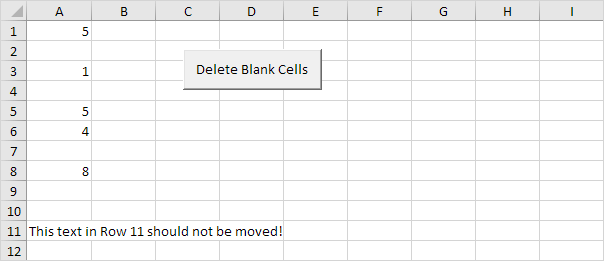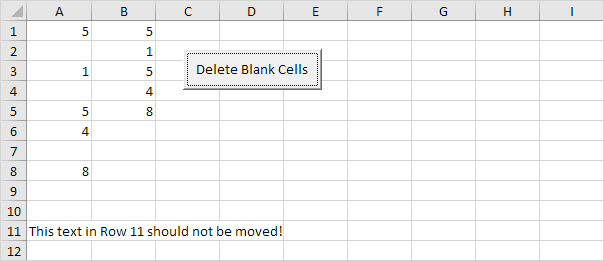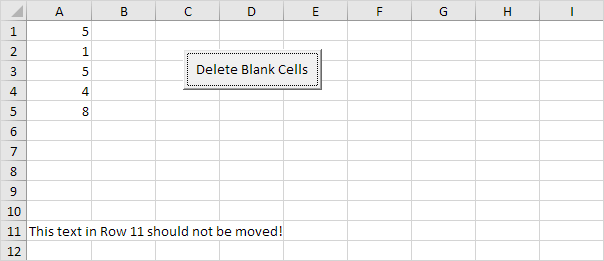# Delete Blank Cells

Below we will look at a program in Excel VBA that deletes blank cells.

Situation:1. First, we declare two variables of type Integer. One named counter and one named i. We initialize the variable counter with value 0.

Dim counter As Integer, i As Integer
counter = 0

2. Next, we check for each cell whether it is empty or not (<> means not equal to). We are using a loop for this. If not empty, we write the value to column B. The counter holds track of the number of cells that have been copied to column B. Each time we copy a value to column B, we increment counter by 1. This piece of the program looks as follows:

For i = 1 To 10
If Cells(i, 1).Value <> "" Then
Cells(counter + 1, 2).Value = Cells(i, 1).Value
counter = counter + 1
End If
Next i

Result so far:3. Finally, we empty Range("A1:A10"), copy the values of column B to column A, and empty Range("B1:B10").

Range("A1:A10").Value = ""
Range("A1:A10").Value = Range("B1:B10").Value
Range("B1:B10") = ""

Result:Go to Next Chapter: Loop